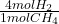how many liters of hydrogen gas I needed to react with CS2 to produce 2.5 L of CH4 At STP

Question

how many liters of hydrogen gas I needed to react with CS2 to produce 2.5 L of CH4 At STP

in progress 0
6 months 2021-07-29T14:29:47+00:00 1 Answers 28 views 0

9.8 L

Explanation:

The reaction that takes place is:

• 4H₂(g) + CS₂(g) → CH₄(g)+ 2H₂S(g)

At STP, 1 mol of any gas occupies 22.4 L.

We calculate how many moles are there in 2.5 L of CH₄ at STP:

• 2.5 L ÷ 22.4 L/mol = 0.11 mol CH₄

Then we convert CH₄ moles into H₂ moles, using the stoichiometric coefficients of the reaction:

• 0.11 mol CH₄ *= 0.44 mol H₂

Finally we calculate the volume that 0.44 moles of H₂ would occupy at STP:

• 0.44 mol * 22.4 L/mol = 9.8 L